Electron. J. Diff. Eqns., Vol. 2001(2001), No. 60, pp. 1-20.

### Solutions of nonlinear parabolic equations without growth restrictions on the data Lucio Boccardo, Thierry Gallouet, & Juan Luis Vazquez

Abstract:
The purpose of this paper is to prove the existence of solutions for certain types of nonlinear parabolic partial differential equations posed in the whole space, when the data are assumed to be merely locally integrable functions, without any control of their behaviour at infinity. A simple representative example of such an equation is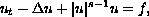which admits a unique globally defined weak solution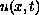if the initial function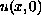is a locally integrable function in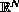,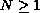, and the second member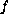is a locally integrable function of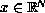and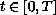whenever the exponentis larger than 1. The results extend to parabolic equations results obtained by Brezis and by the authors for elliptic equations. They have no equivalent for linear or sub-linear zero-order nonlinearities.

Submitted July 17, 2001. Published September 12, 2001.
Math Subject Classifications: 35K55, 35K65.
Key Words: Nonlinear parabolic equations, global existence, growth conditions.

Show me the PDF file (413K), TEX file, and other files for this article.Lucio Boccardo Dipartimento di Matematica, Universita di Roma 1 Piazza A. Moro 2, 00185 Roma, Italy e-mail: boccardo@mat.uniroma1.it Thierry Gallouet CMI, Universite de Marseille I 13453, France e-mail: gallouet@cmi.univ-mrs.fr Juan Luis Vazquez Departamento de Matematicas Universidad Autonoma de Madrid 28049 Madrid, Spain e-mail: juanluis.vazquez@uam.es